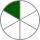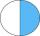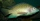# Fractions - 6th grade (11y) - math problems

#### Number of examples found: 250

• Reduce to lowest termsReduce to lowest terms : 32/124
• Sum of fractionsWhat is the sum of 2/3+3/5?
• Ratio 11Simplify this ratio 10 : 1/4
• Missing numberBlank +1/6 =3/2 find the missing number
• Fractions 4How many 2/3s are in 6?
• FractionDetermine for what x fraction ?:
• Mixed2improperWrite the mixed number as an improper fraction. 166 2/3
• One sixthHow many sixths are two thirds?
• Reciprocal equation 3Solve reciprocal equation: 1/2 + 2/3=1/x
• EquationSolve equation and check the result: 1.4x - 3/2 + x - 9,8 = x + 0,4/3 - 7 + 1,6/6
• To improper fractionChange mixed number to improper fraction a) 1 2/15 b) -2 15/17
• Simple equation 5Solve equation with fractions: X × 3/8 = 1/2
• Simple equation 2Find X in this simple equation: X/9 = 96/108
• DenominatorCalculate the missing denominator x: ?
• Product of two fractionsProduct of two fractions is 9 3/5 . If one of the fraction is 9 3/7. Find the other fraction.
• In fractionsAn ant climbs 2/5 of the pole on the first hour and climbs 1/4 of the pole on the next hour. What part of the pole does the ant climb in two hours?
• Evaluate expressionCalculate the value of the expression z/3 - 2 z/9 + 1/6, for z = 2
• Cake 71/3 of a cake shared with 4 people. What share of the whole cake has each people?
• Unknown number 6Determine x if 1/6 of x is equal to 2/5 of the number 24.
• TilapiaA fish vendor sells 5/7 kilos of tilapia for 73.50. If you will buy 2 1/7 kilos of tilapia, how much will it cost?

Do you have an interesting mathematical word problem that you can't solve it? Submit a math problem, and we can try to solve it.

We will send a solution to your e-mail address. Solved examples are also published here. Please enter the e-mail correctly and check whether you don't have a full mailbox.

Please do not submit problems from current active competitions such as Mathematical Olympiad, correspondence seminars etc...
Need help calculate sum, simplify or multiply fractions? Try our fraction calculator.